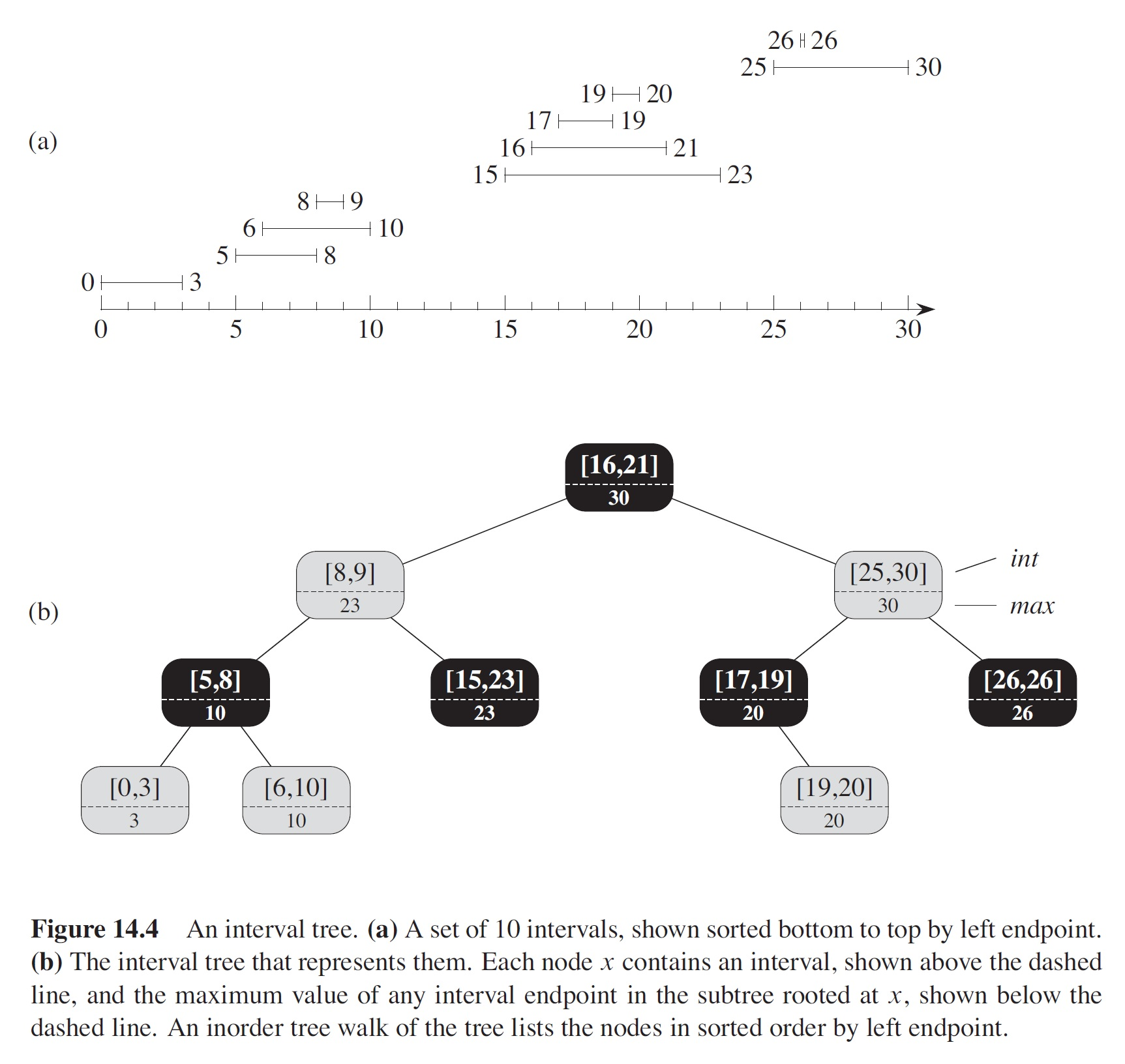CIS 5511. Programming Techniques

Self-balancing BST

Since in Binary Search Trees (BSTs) all major operations have run-time proportional to the height of the tree, a "balanced" BST will be close to the best case, in which each node has two subtrees with similar heights. However, to keep this balance, structure adjustments are needed after certain insertions and deletions. Self-balancing Binary Search Trees are BSTs with this capability. There are several data structures of this type.

### 1. AVL tree

For any node in an AVL tree, the height of the left subtree and right subtree differ by 1 at most.

The balance factor of a node is hL − hR (or hR − hL — it does not matter as far as used consistently) where hL is the height of its left subtree and hR is the height of its right subtree. In an AVL tree, valid balance factors are +1, -1, or 0.

After an insertion or deletion, the balance factor of some node may become +2 or -2, then the tree is "rebalanced" to restore its balance by moving some nodes around.

Let's start with BSTs with 3 nodes. There are 5 possibilities, but only one of the them is balanced.

Example: each node shows its key over its balance factor

```                C/2
/
B/1
/
A/0
```
This is considered a "LL" situation, as the first unbalanced node (C/2) is left heavy (+2) and so is its left child (+1).

To rebalance the tree without changing the relative order of nodes (as defined in binary search tree), we can use a rotation.

The result of a right rotation would be

```           B/0
/  \
A/0  C/0
```
Another example:
```             C/2
/
A/-1
\
B/0
```

This is a "LR" situation, as the unbalanced node is "left heavy" (+2), and its left child is "right heavy" (-1). Result of first a left and then a right rotation would be: After left rotation of bottom 2 nodes:

```             C/2
/
B/1
/
A/0
```

which is the "LL" case, then after a right rotation the tree is balanced

```           B/0
/  \
A/0  C/0
```

There are two mirror-image situations: "RR" and "RL".

Now we can extend the above simple cases into general situations, where the nodes to be rotated have subtrees. The rotations should keep the order of all the nodes involved.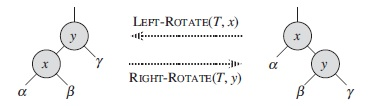The left rotation algorithm: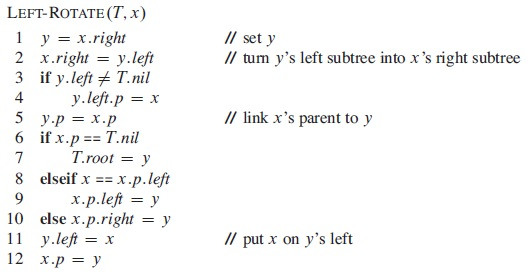The algorithm is used in the following tree: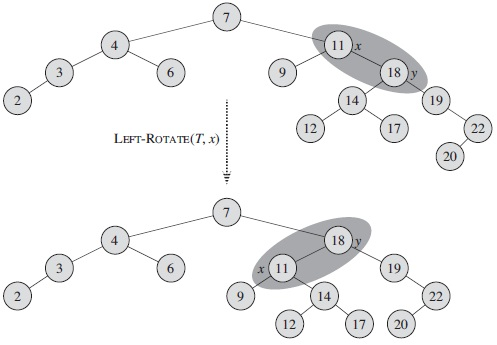The right rotation algorithm is symmetric.

After an insertion or deletion, one or more "out-of-balance" nodes (with balance factor 2 or -2) may appear in the path from root to the insertion/deletion location, but cannot show up outside the path. If we check the "out-of-balance" node that is farest from the root, there are only 4 canonical forms.

LL case:

```              C/2
/   \
B/1     CR
/   \
A/0     BR
/   \
AL     AR
```

Here AL, AR, BR, and CR have the same height.

From properties of binary search trees, we know the following: AL < A < AR < B < BR < C < CR

This requires a right rotation. B will become the root. Its right subtree will be C. C will no longer have B as its right subtree but will have BL instead. B and C have balance factors of 0. The other nodes balance factors remain the same as before.

```          B/0
/   \
A/0     C/0
/  \    /   \
AL    AR  BR   CR
```
where we still have the order AL < A < AR < B < BR < C < CR.

LR Case:

```               C/2
/   \
A/-1     CR
/    \
AL     B/0
/   \
BL    BR
```
Assume that all the subtrees, AL, BL, BR, and CR have the same height. For the order among the nodes, here we have: AL < A < BL < B < BR < C < CR.

A left rotation (around A) gives us:

```              C/2
/   \
B/1     CR
/   \
A/0    BR
/   \
AL    BL
```

then a right rotation (around C) gives us a balanced tree:

```          B/0
/   \
A/0     C/0
/   \   /   \
AL    BL BR   CR
```
where we still have AL < A < BL < B < BR < C < CR.

The RL and RR cases are symmetric.

These four cases cover all possibilities. For an out-of-balance node (i.e., with balance factor 2 or -2), if its child on the heavy side has balance factor 1 or -1, it directly maps into one of the above four cases: 2/1 to LL, 2/-1 to LR, -2/-2 to RR, and -2/1 to RL. Please note that if the third node (A in LL, B in LR and RL, and C in RR) has a balance factor 1 or -1, we can still use the above procedure to rebalance the tree. If the heavy-side child has balance factor 0 (which can only happen after a deletion, but not after an insertion), it is treated as LL or RR, and the tree will be balanced.

An AVL tree is maintained by making some changes to the algorithm for BST insertion and deletion. We can visualize the change as retracing the path we took to do the insertion/deletion and checking to see whether the change caused a node to become unbalanced. If necessary, a rebalance action is taken.

In a balanced BST, the time complexity of search, insertion, and deletion is O(log n), so it is more efficient than BTS and linear data structures (array and linked list).

### 2. Red-Black Tree

Red-Black tree is another self-balancing BST. It has a lower standard for "balance" (so is more efficient), though less intuitive than AVL tree.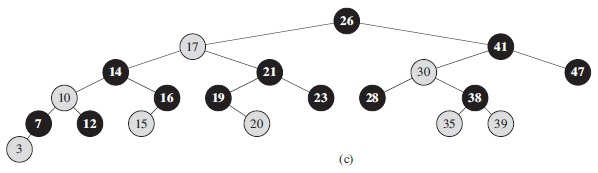A Red-Black tree has the following properties:

1. A node is either red or black.
2. The root is black.
3. If a node is red, then its children are both black (an empty reference, null, is considered to refer to a black node).
4. Every path from a node to a descendant leaf contains the same number of black nodes.
It follows from the above definition that in a Red-Black tree, no leaf is more than twice as far from the root as any other, which gives another type of "balance". A red-black tree with n internal nodes has height at most 2log(n+1).
```                11
/    \
2       14
/   \
1     7
/   \
5     8
```
Similar to in AVL tree, an insertion in a Red-Black tree is an insertion in a binary search tree, sometimes followed by a rotation to keep the balance.

The new node gets the color red. After the insertion, there are the following possibilities:

1. If the parent of the new node is black, the operation ends.
2. If the parent and its sibling are also red, the color of the parent, its sibling, and the grandparent are all changed. If the grandparent is the root, it is changed back to black.
3. If the parent is red, but has no red sibling, do a rotation among the new node, its parent, and its grandparent, so that the node with a middle value of the three takes the previous position of the grandparent (in the same way as in an AVL tree), and make it black and the other two red.
The above process may need to be repeated for the grandparent node, all the way up the tree.

For deletion in a Red-Black tree, do binary search tree deletion first, then check the balance. Since the node actually removed has one or no child, there are several possibilities. If the removed node is red, the process ends. However, if the removed node is black, rotation and re-coloring may be necessary.

Since deletion is complicated in Red-Black tree, sometimes the node is just marked as "deleted", without being removed from the tree.

In a Red-Black tree, the major operations take O(log N) time in the worst cases.

The Java TreeMap class is based on a Red-Black tree.

### 3. Augmenting Data Structures

Red-Black tree can be augmented to support additional operations. These additional attributes must be maintained in the operations.

One attribute that can be added into each node is the number of nodes in the subtree rooted at the node (including itself), that is, the size of the subtree. Using this information, An order-statistic tree can be built, in which algorithm OS-SELECT(x, i) retrieves an element with a given rank.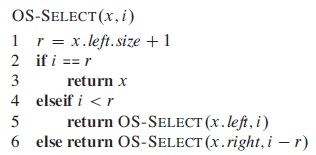Algorithm OS-RANK(T, x) calculates the rank of a given node by counting the nodes "on its left".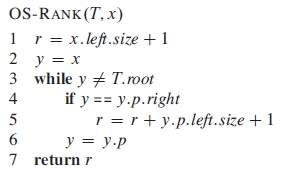Red-black trees can also be augmented to support operations on dynamic sets of intervals, which can be open or closed. The key of each interval x is the low endpoint, x.int.low, of the interval. In addition to the intervals themselves, each node x contains a value x.max, which is the maximum value of any interval endpoint stored in the subtree rooted at x. Algorithm INTERVAL-SEARCH(T,i) searches the tree for the given interval.